Next: Worked example 9.1: Angular Up: Angular momentum Previous: Angular momentum of an

## Angular momentum of a multi-component system

Consider a system consisting ofmutually interacting point particles. Such a system might represent a true multi-component system, such as an asteroid cloud, or it might represent an extended body. Let the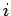th particle, whose mass is, be located at vector displacement. Suppose that this particle exerts a forceon theth particle. By Newton's third law of motion, the forceexerted by theth particle on theth is given by(437)

Let us assume that the internal forces acting within the system are central forces--i.e., the force, acting between particlesand, is directed along the line of centres of these particles. See Fig. 87. In other words,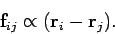(438)

Incidentally, this is not a particularly restrictive assumption, since most forces occurring in nature are central forces. For instance, gravity is a central force, electrostatic forces are central, and the internal stresses acting within a rigid body are approximately central. Suppose, finally, that theth particle is subject to an external force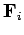.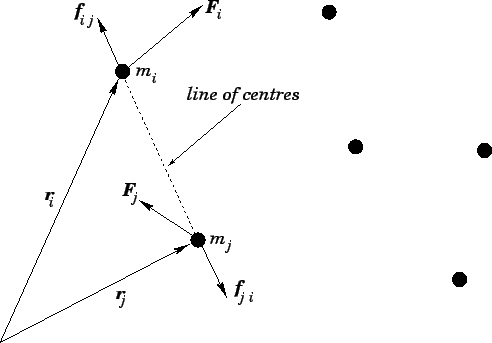The equation of motion of theth particle can be written(439)

Taking the vector product of this equation with the position vector, we obtain(440)

Now, we have already seen that(441)

We also know that the total angular momentum,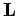, of the system (about the origin) can be written in the form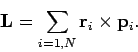(442)

Hence, summing Eq. (440) over all particles, we obtain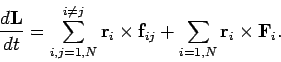(443)

Consider the first expression on the right-hand side of Eq. (443). A general term,, in this sum can always be paired with a matching term,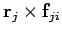, in which the indices have been swapped. Making use of Eq. (437), the sum of a general matched pair can be written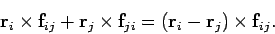(444)

However, if the internal forces are central in nature thenis parallel to. Hence, the vector product of these two vectors is zero. We conclude that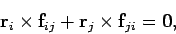(445)

for any values ofand. Thus, the first expression on the right-hand side of Eq. (443) sums to zero. We are left with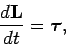(446)

where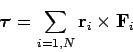(447)

is the net external torque acting on the system (about an axis passing through the origin). Of course, Eq. (446) is simply the rotational equation of motion for the system taken as a whole.

Suppose that the system is isolated, such that it is subject to zero net external torque. It follows from Eq. (446) that, in this case, the total angular momentum of the system is a conserved quantity. To be more exact, the components of the total angular momentum taken about any three independent axes are individually conserved quantities. Conservation of angular momentum is an extremely useful concept which greatly simplifies the analysis of a wide range of rotating systems. Let us consider some examples.Suppose that two identical weights of massare attached to a light rigid rod which rotates without friction about a perpendicular axis passing through its mid-point. Imagine that the two weights are equipped with small motors which allow them to travel along the rod: the motors are synchronized in such a manner that the distance of the two weights from the axis of rotation is always the same. Let us call this common distance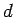, and letbe the angular velocity of the rod. See Fig. 88. How does the angular velocitychange as the distanceis varied?

Note that there are no external torques acting on the system. It follows that the system's angular momentum must remain constant as the weights move along the rod. Neglecting the contribution of the rod, the moment of inertia of the system is written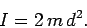(448)

Since the system is rotating about a principal axis, its angular momentum takes the form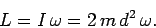(449)

Ifis a constant of the motion then we obtain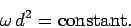(450)

In other words, the system spins faster as the weights move inwards towards the axis of rotation, and vice versa. This effect is familiar from figure skating. When a skater spins about a vertical axis, her angular momentum is approximately a conserved quantity, since the ice exerts very little torque on her. Thus, if the skater starts spinning with outstretched arms, and then draws her arms inwards, then her rate of rotation will spontaneously increase in order to conserve angular momentum. The skater can slow her rate of rotation by simply pushing her arms outwards again.

Suppose that a bullet of massand velocity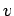strikes, and becomes embedded in, a stationary rod of mass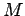and length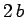which pivots about a frictionless perpendicular axle passing through its mid-point. Let the bullet strike the rod normally a distancefrom its axis of rotation. See Fig. 89. What is the instantaneous angular velocityof the rod (and bullet) immediately after the collision?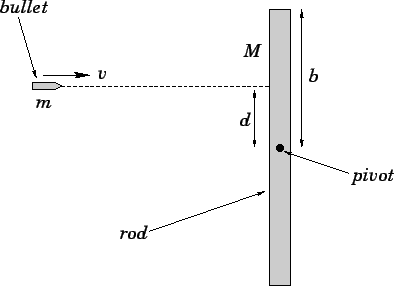Taking the bullet and the rod as a whole, this is again a system upon which no external torque acts. Thus, we expect the system's net angular momentum to be the same before and after the collision. Before the collision, only the bullet possesses angular momentum, since the rod is at rest. As is easily demonstrated, the bullet's angular momentum about the pivot point is(451)

i.e., the product of its mass, its velocity, and its distance of closest approach to the point about which the angular momentum is measured--this is a general result (for a point particle). After the collision, the bullet lodges a distancefrom the pivot, and is forced to co-rotate with the rod. Hence, the angular momentum of the bullet after the collision is given by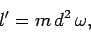(452)

whereis the angular velocity of the rod. The angular momentum of the rod after the collision is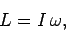(453)

where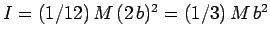is the rod's moment of inertia (about a perpendicular axis passing through its mid-point). Conservation of angular momentum yields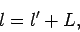(454)

or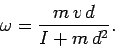(455)Next: Worked example 9.1: Angular Up: Angular momentum Previous: Angular momentum of an
Richard Fitzpatrick 2006-02-02